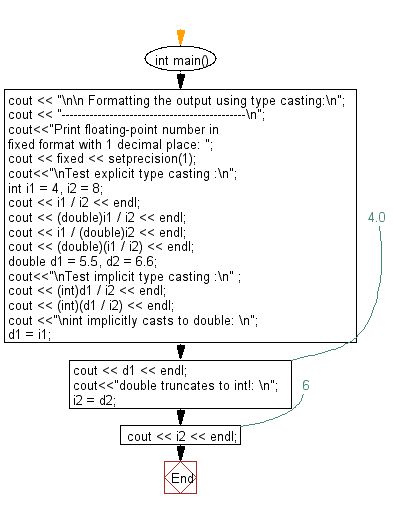﻿ C++ Exercises: Test the Type Casting - w3resource

# C++ Exercises: Test the Type Casting

## C++ Basic: Exercise-36 with Solution

Write a program in C++ to test Type Casting.

Sample Solution:

C++ Code :

``````#include <iostream>
#include <iomanip>
using namespace std;

int main()
{

cout << "\n\n Formatting the output using type casting:\n";
cout << "----------------------------------------------\n";
cout<<"Print floating-point number in fixed format with 1 decimal place: ";
cout << fixed << setprecision(1);
cout<<"\nTest explicit type casting :\n";
int i1 = 4, i2 = 8;
cout << i1 / i2 << endl;
cout << (double)i1 / i2 << endl;
cout << i1 / (double)i2 << endl;
cout << (double)(i1 / i2) << endl;

double d1 = 5.5, d2 = 6.6;
cout<<"\nTest implicit type casting :\n" ;
cout << (int)d1 / i2 << endl;
cout << (int)(d1 / i2) << endl;
cout <<"\nint implicitly casts to double: \n";
d1 = i1;
cout << d1 << endl;  // 4.0
cout<<"double truncates to int!: \n";
i2 = d2;
cout << i2 << endl;  // 6
}
``````

Sample Output:

```Formatting the output using type casting:
----------------------------------------------
Print floating-point number in fixed format with 1 decimal place:
Test explicit type casting :
0
0.5
0.5
0.0

Test implict type casting :
0
0

int implicitly casts to double:
4.0
double truncates to int!:
6
```

Flowchart:C++ Code Editor:

What is the difficulty level of this exercise?

﻿

## C++ Programming: Tips of the Day

Why is there no std::stou?

The most pat answer would be that the C library has no corresponding "strtou", and the C++11 string functions are all just thinly veiled wrappers around the C library functions: The std::sto* functions mirror strto*, and the std::to_string functions use sprintf.

Ref: https://bit.ly/3wtz2qA

We are closing our Disqus commenting system for some maintenanace issues. You may write to us at reach[at]yahoo[dot]com or visit us at Facebook